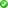/
/
/
11The specific cost of each source of financing the after-tax
Not my Question
Flag Content

# Question : 11The specific cost of each source of financing the after-tax : 1405658

11The specific cost of each source of financing is the after-tax cost of obtaining the financing using the historically based cost reflected by the existing financing on the firm's books.
TrueFalse

12In general, flotation costs include two components, underwriting costs and administrative costs.True
False

13Flotation costs reduce the net proceeds from the sale of a bond whether sold at a premium, at a discount, or at its par value.True
False

14The net proceeds used in calculation of the cost of long-term debt are funds actually received from the sale after paying for flotation costs and taxes.
TrueFalse

15Since preferred stock is a form of ownership, the stock will never mature.True
False

16Preferred stock represents a special type of ownership interest in the firm and, thus, the preferred stockholders must receive their stated dividends prior to the distribution of any earnings to common stockholders and bondholders.
TrueFalse

17When the net proceeds from the sale of a bond equal its par value, the beforetax cost would just equal the coupon interest rate.True
False

18The amount of preferred stock dividends that must be paid each year may be stated in dollars (i.e., x-dollar preferred stock) or as a percentage of the firm's earnings (i.e., x-percent preferred stock).
TrueFalse

19The cost of preferred stock is typically higher than the cost of long-term debt (bonds) because the cost of long-term debt (interest) is tax deductible.True
False

20The cost of common stock equity may be measured using either the constant growth valuation model or the capital asset pricing model.